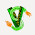## Tuesday, November 8, 2011

### Models for discrete metric spaces

It is good to have random models to test algorithms.

For graph algorithms, the G(n,p) model is like an old friend. For network algorithms, the analog is the complete graph with i.i.d. exponentially distributed edge weights. For algorithms on discrete metric spaces, what's a good model for a random discrete metric space?

Is there a model such that the marginal distribution of the distance between any pair of vertices has an exponential distribution? If so, then what would be a maximum entropy distribution that would have those marginals?

1.You can certainly take a single exponential E and declare that every pair of vertices is at distance exactly E.

When n=3 it already isn't obvious (to me) that you can do better. In that case you are looking for a triple (E_1,E_2,E_3) such that each coordinate is exponentially distributed and such that E_1 < E_2+E_3, E_2 < E_3+E_1, and E_3 < E_1+ E_2, so that the triangle inequality holds. Can this be done?

Maybe Mathoverflow could help.

2.A less constructive, but maybe more noninformative (and therefore close to max entropy) way would be to sample from the metric cone ? you'd have to normalize but you'd do that anyway, and then you'd end up with a set of constraints defining a polytope which can be sampled from using a polytope sampling method.

3.4.Why is this post always the first when I visited feedworld.net/toc?

5.Because it is the most important theory post in history. Period.

6.There's a problem with your blog!!
On TCS aggregator it appears permanently as the most recent blog update, even when it's not.
Please, someone, fix this!
I understand that it might not be the responsibility of Claire, and it might be a bug in the TCS aggregator site.

7.not Claire's faultFebruary 1, 2012 at 8:07 AM

Is the date correct---was this actually posted Nov. 8, 2011? Seems to be so, since the first two comments are dated from then.

If so, it only popped up quite recently as the top spot on the aggregator, so the problem is presumably on the aggregator side and not some quirk of the individual blog.

8.Some time ago my blog was removed from the aggregator because, for some unknown reason, every post (not just the scientific TCS posts) was appearing there. A few days ago I was told that this had been fixed and that it was on the aggregator again. It appears that there now is another problem. I'll bring this to Arvind's attention.

Note: Only a member of this blog may post a comment.